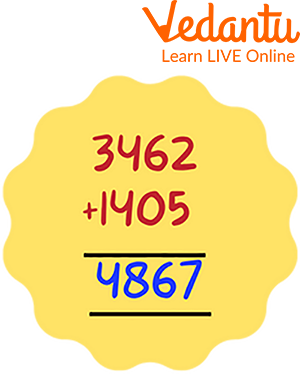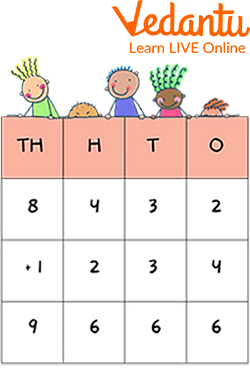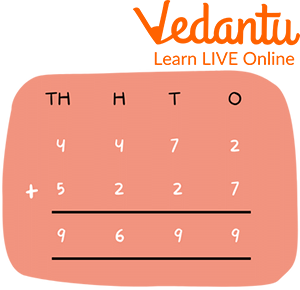Courses
Courses for Kids
Free study material
Free LIVE classes
MoreLIVE
Join Vedantu’s FREE Mastercalss

## An Introduction to 4-Digit Addition

Addition is one of the most prime and basic arithmetical operations which is used in Mathematics. It depicts a process of adding two or more quantities together to get the required total. The numbers being added to each other are called addends and the result obtained after addition is called the sum. Here, in this article, we will be discussing the topic of addition and sums related to addition without regrouping. So, let us begin with our learning.

## What is Regrouping in Math?

Regrouping in Maths is defined as the process of making a group of tens while performing operations, basic addition, and subtraction. It comes into use when we work with double-digit numbers.

## What is Addition without Regrouping?

Addition without regrouping is the process of performing the addition of two numbers corresponding to their place values without taking into consideration the rearrangement of numbers. It is carried out when the digits of the numbers add up to obtain the result that is nine or less. No carrying of tens or hundreds is allowed here. Regrouping and carrying have the same meanings when discussing the addition of integers.## Steps to Solve 4-Digit Addition without Regrouping

When we tend to add the two numbers, we always start adding the numbers from the right-hand side and then move to the left-hand side. Steps followed to add without regrouping are given below:

• Arrange the given numbers according to their place values in the column format.

• The place values should fall in the same columns when you put the addends one on top of the other i.e. ones of the number in the one's place, tens in the tens place, hundreds in the hundreds place, and thousands in the thousands place.

• Separately add up each column, starting from the first position at the right.

• Continue the above steps till the addition does not takes place at each position.

• The totals of each column should appear below each column and the line.

## Solved Examples

1. Add without regrouping: 8432 and 1234.

Ans: Steps to be followed to add without regrouping are given below:

• Arrange the given numbers 8432 and 1234 according to their place values in column format.

• The place values should fall in the same columns when you put the addends one on top of the other i.e. ones of the number in the one's place, tens in the tens place, hundreds in the hundreds place, and thousands in the thousands place.

• Separately add up each column, starting from the first position at right i.e. 2 and 4.

• Continue the above steps till the addition does not takes place at all the four positions.

• The totals of each column should appear below each column and below the line i.e. 9666.

Thus, the result of 4-digit addition without regrouping is 9666.Solved Example of Addition without Regrouping

2. Add up the given quantities: 4472 and 5227.

Ans: Steps to be followed to add without regrouping are given below:

• Arrange the given numbers 4472 and 5227 according to their place values in column format.

• The place values should fall in the same columns when you put the addends one on top of the other i.e. ones of the number in the one's place, tens in the tens place, hundreds in the hundreds place, and thousands in the thousands place.

• Separately add up each column, starting from the first position at right i.e. 2 and 7.

• Continue the above steps till the addition does not takes place at all four positions.

• The totals of each column should appear below each column and below the line i.e. 9699.

Thus, the result of addition without regrouping is 9699.Solved Example of 4-digit Addition without Regrouping

## Practice Questions

Solve the following by adding without regrouping:

1. 4824 + 3145

Ans: 7969

2. 7454 + 3532

Ans: 10986

3. 3287 + 5711

Ans: 8998

4. 4374 + 2603

Ans: 6977

5. 1209 + 6480

Ans: 7689

6. 7465 + 2323

Ans: 9788

## Summary

This article has covered all the details about the addition of two 4-digit numbers and some solved examples based on the addition without regrouping, using eye-catching pictures to draw students' attention in understanding the new topic. Addition without regrouping is quite simpler because it does not deal with carrying problems. Some practice questions are being assigned to the students for a better understanding of the concepts.

Last updated date: 03rd Oct 2023
Total views: 97.5k
Views today: 2.97k

## FAQs on 4-Digit Addition without Regrouping

1. Which is the smallest and largest possible 4-digit number?

The smallest 4-digit number is 1000 and the greatest 4-digit number is 9999. There are a total of 9000 numbers of 4 digits.

2. What is Commutative Law for an addition?

The commutative law of addition states that the addition of two numbers is not affected by their order i.e. when we add two numbers, the result will always be equal to the addition of their interchanged positions.

A + B = B + A

For Example, 1 + 2 = 3 = 2 + 1

4 + 5 = 9 = 5 + 4

3. Can repeated addition be used in place of multiplication?

Yes, repeated addition can be used in place of multiplication because both terms mean the same. For example: if we need to multiply 4 with 3, then we can also express this multiplication as the repeated addition of 4, three times, i.e.,  4 × 3 ~ 4 + 4 + 4 = 12.